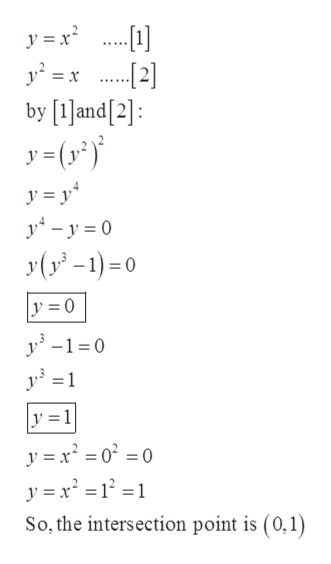# Using disks or washers, find the volume of the solid obtained by rotating the region bounded by the curves y=x2 and y2=x about the x-axis.

Question
18 views

Using disks or washers, find the volume of the solid obtained by rotating the region bounded by the curves y=x2 and y2=x about the x-axis.

check_circle

Step 1

Given:

Step 2

Find the intersection point:help_outlineImage Transcriptionclose. y² =x .  by and: y=(r*} y = x? ..... y = y* y* – y = 0 У(у - 1) -о y = 0 y -1=0 y =1 %3D y =1 y = x = 0° = 0 y = x² = 1° =1 So, the intersection point is (0, 1) fullscreen
Step 3

Graph:

...

### Want to see the full answer?

See Solution

#### Want to see this answer and more?

Solutions are written by subject experts who are available 24/7. Questions are typically answered within 1 hour.*

See Solution
*Response times may vary by subject and question.
Tagged in

### Integration